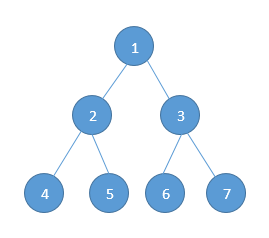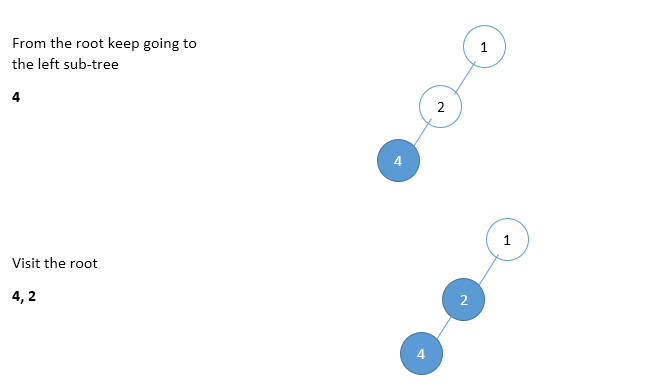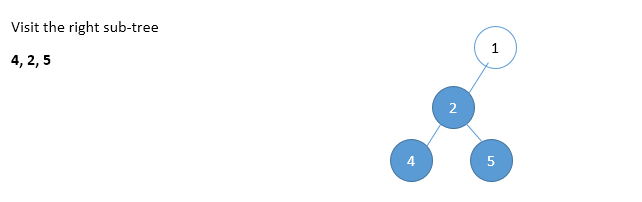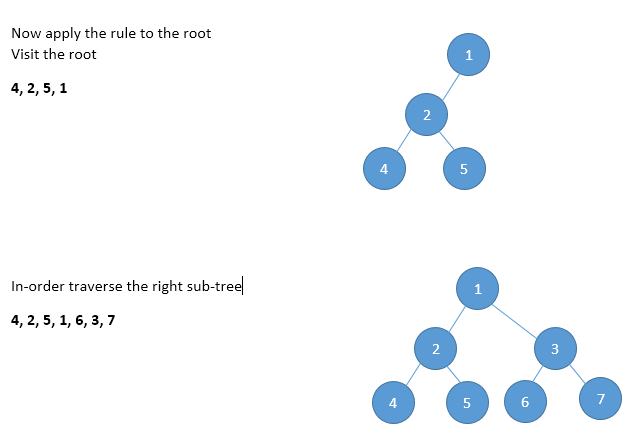Home Theory InOrder Traversal in a binary tree

# InOrder Traversal in a binary tree

0 comment

We discussed about the tree traversal techniques in this post-

Please see pre-order traversal to understand the basics of visiting nodes. We have our same sample treeNow let us try to understand the In-order traversal. In in-order traversal, the root is traversed between the sub trees. In-order traversal is defined as follows.

• Traverse the left sub-tree
• Visit the node
• Traverse the right sub-tree

So when we apply the in-order traversal on our example tree this will be done as:-We get the final in-order traversal as:- 4, 2, 5, 1, 6, 3, 7

The recursive version of in-order traversal can be written as:-

void inOrderRecursive(struct binaryTreeNode * root)
{
if(root)
{
// In-order traversal of left sub-tree (Step 1)
inOrderRecursive(root -> left);

// Visit the root (Step 2)
printf("%d ", root -> data);

// In-order traversal of right sub-tree(Step 3)
inOrderRecursive(root -> right);
}
}


### Non-Recursive In-order Traversal

Non-recursive version of In-order traversal us very much similar to Pre-order. The only change is instead of processing the node before going to left sub-tree, process it after popping (which indicates after completion of left sub-tree processing).

void inOrderNonRecursive(struct binaryTreeNode * root)
{
// Create a new empty stack
struct stackNode * S = NULL;

// We need to loop until the stack is empty
while(1)
{
// If the root is not null
while(root)
{
// Push the first element onto the stack
S = push(S, root);

// Go to left sub-tree and keep on adding it to the stack
root = root -> left; // (Step 1)
}

// Check if the stack is empty
if(isStackEmpty(S))
break;

// else we need to pop out the element
root = S -> data;

// Remove the element from the stack
S = S -> next;

// After popping process the current node
printf("%d ",root -> data); // Step 2

// After printing, left sub-tree and root have been completed
// Go to the right sub-tree
root = root -> right; // Step 3
}

// We need to delete the stack
free(S);
}


Time Complexity:- O(n)
Space Complexity:- O(n)

#### You may also like

This website uses cookies to improve your experience. We'll assume you're ok with this, but you can opt-out if you wish. Accept Read More